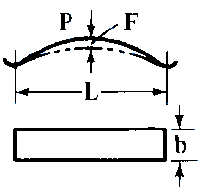Related Resources: calculators

### Flat Spring Supported Both Ends Equations and Calculator

Beam Stress Deflection Equations and Calculators
Spring Design Equations and Calculators

Flat spring design equations and calculator: This spring is often used to overcome operating space limitations in various products such as electric switches and relays. The equations are based on standard beam formulas where the deflection is small.

Flat spring supported on both ends design equations and calculator.Deflection:

eq. 1
F = P L3 / 4 E b t3

eq. 2
F = Sb L2 / 6 E t

eq. 3
P = 2 Sb b t2 / 3 L

eq. 4
P = 4 E b t3 F / L3

Stress, Bending:

eq. 5
Sb = 3 P L / 2 b t^2

eq. 6
Sb = 6 E t F / L2

Thickness, Spring:

eq. 7
t = Sb L2 / 6 E F

eq.8

 t =Where:

L = Active length subject to deflection (inches),
b = Widest width of spring (in),
E = Modulus of Elasticity in Tension, (lbs/in2),
t = Thickness, (in),
Sb = Stress, bending (lbs/in2),
F - Deflection, for on active section (in)